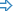Scripts may often need to write data (e.g. test output) to a file. The data file may be kept for reference as a standard, or compared against an existing standard output file (see About File Checkpoints).

In DelphiScript, file operations are supported by specific functions built into TestComplete (see DelphiScript Routines). For JavaScript, JScript, Python, VBScript, C#Script and C++Script the situation is a bit different. These languages do not include functions to write or read data files. One possible solutions, as the examples show, is to call on the Windows Scripting Host to create a file object via OLE and then use this object to work with the file (see the documentation on the `File` object in the MSDN Library). Another solution is to use functions provided by the aqFile object that is available in all supported scripting languages.

Other topics that illustrate how to work with files in scripts:Processing Files in a FolderGetting File SizeReading Text FilesWorking With XML Files From Scripts

For information on how to compare files, see About File Checkpoints.

• Creating a text file and writing a string into it through DelphiScript routines

DelphiScript

procedure Test;
var
f, s : OleVariant;
begin
s := 'Hello, world!';
AssignFile(f, 'c:\MyFile.txt');
Rewrite(f);
Write(f, s);
CloseFile(f);
end;

• Creating a text file and writing a string into it through FileSystemObject

JavaScript

function Test()
{
const ForReading = 1, ForWriting = 2, ForAppending = 8, TristateFalse = 0;
var fs, f, sPath;
sPath = "c:\\MyFile.txt";
// Creates a new file object
fs = getActiveXObject("Scripting.FileSystemObject");

if (!fs.FileExists(sPath))
{
objFile = fs.CreateTextFile(sPath);
objFile.Close();
}
f = fs.OpenTextFile(sPath, ForAppending, TristateFalse);
f.Write("Hello, world!\n");
f.Close();
}

JScript

function Test()
{
var ForReading = 1, ForWriting = 2, ForAppending = 8, TristateFalse = 0;
var fs, f, sPath;
sPath = "c:\\MyFile.txt";
// Creates a new file object
fs = Sys.OleObject("Scripting.FileSystemObject");
// Note that you can also create the FileSystemObject
// using the following code:
// fs = new ActiveXObject("Scripting.FileSystemObject")
if (!fs.FileExists(sPath))
{
objFile = fs.CreateTextFile(sPath);
objFile.Close();
}
f = fs.OpenTextFile(sPath, ForAppending, TristateFalse);
f.Write("Hello, world!\n");
f.Close();
}

Python

``````def Test():
ForWriting = 2
ForAppending = 8
TristateFalse = 0
sPath = "c:\\MyFile.txt"
# Creates a new file object
fs = Sys.OleObject["Scripting.FileSystemObject"]
# Note that you can also create the FileSystemObject
# using the following code:
# fs = new ActiveXObject("Scripting.FileSystemObject")
if not fs.FileExists(sPath):
objFile = fs.CreateTextFile(sPath)
objFile.Close()
f = fs.OpenTextFile(sPath, ForAppending, TristateFalse)
f.Write("Hello, world!\n")
f.Close()``````

VBScript

Sub Test
Const ForReading = 1, ForWriting = 2, ForAppending = 8, TristateFalse = 0
Dim fs, f
sPath = "c:\MyFile.txt"
' Creates a new file object
Set fs = CreateObject("Scripting.FileSystemObject")
If Not fs.FileExists(sPath) Then
Set objFile = fs.CreateTextFile(sPath)
objFile.Close
End If
Set f = fs.OpenTextFile(sPath, ForAppending, TristateFalse)
f.Write "Hello, world!" & vbCrlf
f.Close
End Sub

DelphiScript

procedure Test;
const
ForWriting = 2;
ForAppending = 8;
TristateFalse = 0;
var
f, sPath, fs, objFile : OleVariant;
begin
sPath := 'c:\MyFile.txt';
// Creates a new file object
fs := Sys.OleObject('Scripting.FileSystemObject');
if not fs.FileExists(sPath) then
begin
objFile := fs.CreateTextFile(sPath);
objFile.Close();
end;
f := fs.OpenTextFile(sPath, ForAppending, TristateFalse);
f.Write('Hello, world!' + #13#10);
f.Close();
end;

C++Script, C#Script

function Test()
{
var ForReading = 1, ForWriting = 2, ForAppending = 8, TristateFalse = 0;
var fs, f, sPath;
sPath = "c:\\MyFile.txt";

// Creates a new file object
fs = Sys["OleObject"]("Scripting.FileSystemObject");
// Note that you can also create the FileSystemObject
// using the following code:
// fs = new ActiveXObject("Scripting.FileSystemObject")

if (!fs["FileExists"](sPath))
{
objFile = fs["CreateTextFile"](sPath);
objFile["Close"]();
}
f = fs["OpenTextFile"](sPath, ForAppending, TristateFalse);
f["Write"]("Hello, world!\n");
f["Close"]();
}

• Creating a text file and writing a string into it through the aqFile services

JavaScript, JScript

function Test()
{
var sPath;
sPath = "c:\\MyFile.txt";

if (!aqFile.Exists(sPath))
aqFile.Create(sPath);
aqFile.WriteToTextFile(sPath, "Hello, world!" + "\r\n", aqFile.ctANSI, false);
}

Python

``````def Test():
sPath = "c:\\MyFile.txt"

if not aqFile.Exists(sPath):
aqFile.Create(sPath)
aqFile.WriteToTextFile(sPath, "Hello, world!" + "\r\n", aqFile.ctANSI, False)``````

VBScript

Sub Test
Dim sPath
sPath = "c:\MyFile.txt"

If Not aqFile.Exists(sPath) Then
Call aqFile.Create(sPath)
End If
Call aqFile.WriteToTextFile(sPath, "Hello, world!" & vbCrlf , aqFile.ctANSI, False)
End Sub

DelphiScript

procedure Test;
var
sPath;
begin
sPath := 'c:\MyFile.txt';

if not aqFile.Exists(sPath) then
aqFile.Create(sPath);
aqFile.WriteToTextFile(sPath, 'Hello, world!' + #13#10, aqFile.ctANSI, false);
end;

C++Script, C#Script

function Test()
{
var sPath;
sPath = "c:\\MyFile.txt";

if (!aqFile["Exists"](sPath))
aqFile["Create"](sPath);
aqFile["WriteToTextFile"](sPath, "Hello, world!" + "\r\n", aqFile["ctANSI"], false);
}

Highlight search results